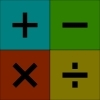You may also likeWorms

Place this "worm" on the 100 square and find the total of the four squares it covers. Keeping its head in the same place, what other totals can you make?Which Scripts?

There are six numbers written in five different scripts. Can you sort out which is which?Highest and Lowest

Put operations signs between the numbers 3 4 5 6 to make the highest possible number and lowest possible number.

One to Fifteen

One to Fifteen

Can you put the numbers from $1$ to $15$ on the circles so that no consecutive numbers lie anywhere along a continuous straight line?

Full screen version

Why do this problem?

This problem is a good one for teaching what consecutive numbers are and for learning to spot them. It is fun to do as long as there are real objects, such as numbered counters, to move around or an interactivity to use.

Sound logical thinking is required but, almost inevitably, some trial and improvement will also be needed!

Possible approach

This photocopiable sheet might be useful but numbered counters will still be necessary.

Key questions

Which circles lie on the fewest straight lines? How might this help?
If you try putting the number $1$ on one circle, where could you put the $2$? Now, where could the $3$ go?
Which numbers have fewer consecutive numbers than the other numbers?
If you have $12$ down, for example, which numbers are consecutive to it?

Possible extension

Learners could be challenged to find as many different solutions they can.

Possible support

Suggest using the interactivity if at all possible as this identifies the consecutive numbers.